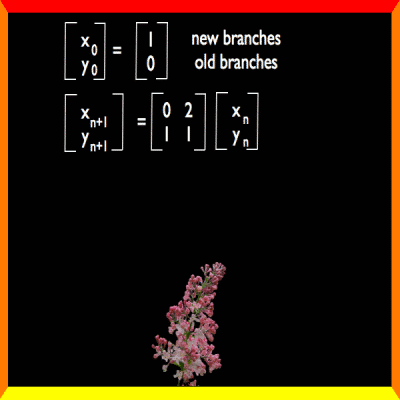M A T H 2 1 B
Mathematics Math21b Spring 2007
Linear Algebra and Differential Equations
Exhibit: lilac bushBretschers book discusses on page 305 the growth of a lilac bush If x(n) is the number of new branches and y(n) is the number of old branches and initially, we have 1 new branch, we want to see how the bush grows if every new branch becomes an old branch in the next step and every old branch additionally grows two new branches. The dynamical system is given by the matrix A with eigenvalues v1,v2 and eigenvalues l1,l2 ```A = | 0 2 | | 1 1 | ``` It has the eigenvalues 2 and -1 and the eigenbasis ```v1= | 1 | v2 = | -2 | | 1 | | 1 | ```